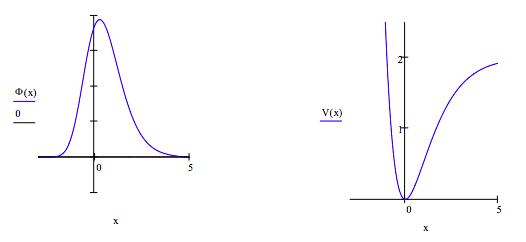Skip to main content

# 423: Variation Method for the Rydberg Potential

## Approximate Methods: The Rydberg Potential

For unit mass the Rydberg potential function has the following energy operator in atomic units.

• Kinetic energy operator: $- \dfrac{1}{2} \frac{d^{2}}{dx^{2}} \blacksquare$
• Potential energy operator: $V(x) = 2 -2 (1 + x) exp(-x)$

Limits of integration: xmin := -3 xmax := 5 $$\int_{x_{min}}^{x_{max}} \blacksquare ~dx$$

Display potential energy:Suggested trial wave function:

$\psi (x, \beta ) = ( \frac{2 \beta}{ \pi})^ \frac{1}{4} exp (- \beta x^{2})$

$\int_{- \infty}^{ \infty} \psi (x, \beta )^{2} dx~assume,~ \beta > 0 \rightarrow 1$

Evaluate the variational energy integral.

$E( \beta ) := \int_{x_{min}}^{x_{max}} \psi (x, \beta ) - \frac{1}{2} \frac{d^{2}}{dx^{2}} \psi (x, \beta ) dx + \int_{x_{min}}^{x_{max}} \psi (x, \beta ) V(x) \psi (x, \beta ) dx$

Minimize the energy with respect to the variational parameter $$\beta$$ and report its optimum value and the ground-state energy.

β := 1 β := Minimize(E, β) β := 0.86327 E(β) = 0.789456

Plot the optimum wave function and the potential energy on the same graph.## Numerical Solution for the Rydberg Potential

Compare the variational result to energy obtained by numerically integrating Schrödinger's equation for the Rydberg potential. For all practical purposes the numerical solution can be considered to be exact.

Numerical integration of Schrödinger's equation:

Given:

$\frac{-1}{2} \frac{d^{2}}{dx^{2}} \Phi (x) + V(x) \Phi (x) = Energy \Phi (x)$

with $$\Phi (x_{min} = 0)$$ and $$\Phi '(x_{min} = 0.1$$

$$\Phi$$ = Odesolve(x, x_{max}) \]

Normalize wave function:

$\Phi (x) := \frac{ \Phi (x)}{ \sqrt{ \int_{x_{min}}^{x_{max}} \Phi (x)^{2} dx}}$

Enter energy guess: Energy = 0.64752Compare the variational and numerical solutions for the Morse oscillator by putting them on the same graph.• Was this article helpful?